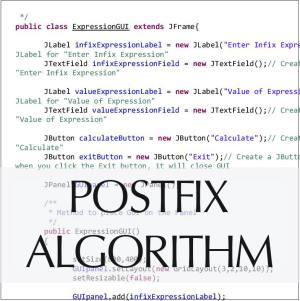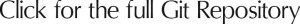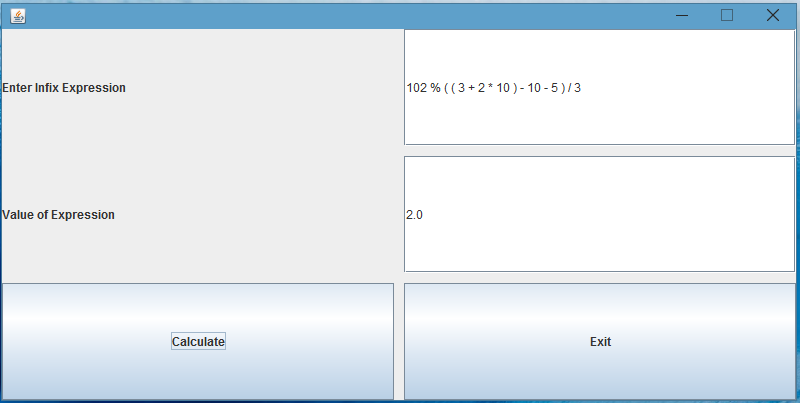# Infix Expression EvaluatorThe Infix Expression Evaluator is a powerful algorithm that takes an arithmetic expression in infix notation, converts it to postfix notation, and evaluates the expression using C-style evaluation. This is a GUI-based interface that allows users to evaluate C-style arithmetic expressions and display their values.

Unlike other programming languages, C does not have a separate Boolean data type, and thus, it has no Boolean expressions. The Infix Expression Evaluator algorithm accommodates this missing Boolean ability by handling Boolean values true and false as non-zero and zero numbers, respectively. The algorithm works with expressions containing numbers, arithmetic and comparison operators, and logical connectives, using only the following operators: comparison operators (<, <=, >, >=, ==, !=), binary logical operators (&&, ||), and binary arithmetic operators (+, -, *, /, %). Positive integer arguments are allowed, and the algorithm assumes C-style evaluation: false is equal to 0, and true is equal to 1 (anything non-zero).

The algorithm is made up of ten files, including ArrayListStack.java, ArrayStack.java, Expression.java, ExpressionEvaluator.java, ExpressionEvaluatorTester.java, ExpressionGUI.java, InfixToPostfixConverter.java, IStack.java, PostfixEvaluator.java, and Token.java.

The InfixToPostfixConverter class is responsible for converting the infix expression to postfix notation. It creates an empty postfix expression, an empty operator stack, and uses temporary local variables to keep track of the tokens. The main loop gets the next token from the infix expression, determines whether it is an operand or an operator, and appends it to the postfix expression or pushes it into the operator stack, respectively. If the next token is a closed parenthesis, the algorithm pulls operators out of the stack and appends them to postfix until it reaches an open parenthesis. If it is an operator, the algorithm compares the precedence of the next token and the top of the stack, and then pushes or pulls operators out of the stack as needed. Finally, it pulls all the operators out of the stack and appends them to postfix.

To demonstrate the Infix Expression Evaluator, we will use a tester to convert and evaluate the following infix expressions: 10 * 5 + 3, 10 * ( 7 + ( 12 – 9 ) ) / 10, and 100 % ( ( 3 + 2 ) + 3 ) / 4. The first expression evaluates to 53, the second to 10, and the third to 1.

The GUI allows users to enter an infix expression manually and compute its value. It is an intuitive way for users to see the algorithm at work and easily evaluate their expressions.